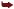The xsl:if Element

The <xsl:if> Element

You use <xsl:if> to make tests and take different actions depending on the result of the test. Its much like the if statement in programming languages. The <xsl:if> element has one attribute:

• test (mandatory). Set to a Boolean (true/false) condition that you want tested .

This element encloses a template body.

Heres how it works: You enclose a template body inside the <xsl:if> element, which tests some expression. If that expression evaluates to true, the template body is used, but if the expression evaluates to false, the template body is ignored:

<xsl:if test="expression">      <!--  template body  -->  </xsl:if>

You can test any XPath expression. Use the following rules for converting them to true/false in the <xsl:if> element:

• If the expression evaluates to a node set, its treated as true if the node set contains at least one node.

• If the expression is a string, its considered true if the string is not empty.

• If the expression is a result tree fragment, its treated as true if it contains any nodes.

• If the expression evaluated to a number, its considered true if its non-zero .

The <xsl:if> element is much like the if-then statement in programming languages. However, there is no <xsl:else> statement that supports if-then-else statementsyou need to use <xsl:choose> instead.

Heres an example. In this case, I list the planets in planets.xml one after the other, and add an HTML horizontal rule, <HR> , element after the last elementbut only after the last element. I can do that with <xsl:if> this way:

Listing 5.1 Using <xsl:if>
<?xml version="1.0"?>  <xsl:stylesheet version="1.0" xmlns::xsl="http://www.w3.org/1999/XSL/Transform">  <xsl:template match="PLANETS">      <HTML>          <HEAD>              <TITLE>                  Planets              </TITLE>          </HEAD>          <BODY>              <xsl:apply-templates select="PLANET"/>          </BODY>      </HTML>  </xsl:template>  <xsl:template match="PLANET">      <P>      <xsl:value-of select="NAME"/>      is planet number <xsl:value-of select="position()"/> from the sun.      </P>      <xsl:if test="position() = last()"><HR/></xsl:if>  </xsl:template>  </xsl:stylesheet>

Here is the result; as you can see, the <HR> element appears after only the last planet has been listed:

<HTML>      <HEAD>          <TITLE>              Planets          </TITLE>      </HEAD>      <BODY>          <P>              Mercury is planet number 1 from the sun.          </P>          <P>              Venus is planet number 2 from the sun.          </P>          <P>              Earth is planet number 3 from the sun.          </P>          <HR>      </BODY>  </HTML>

Heres another example. This is an XML-to-XML transformation, and in it, I list the planets from planets.xml. However, I dont want to have the output simply say, The first three planets are: Mercury Venus Earth, but rather The first three planets are: Mercury, Venus, and Earth. I can add the correct punctuation using the position function to determine which element were working on, and use <xsl:if> to check the position:

Listing 5.2 Second Example Using <xsl:if>
<?xml version="1.0"?>  <xsl:stylesheet version="1.0"  xmlns:xsl="http://www.w3.org/1999/XSL/Transform">  <xsl:output method="xml"/>  <xsl:template match="PLANETS">  <DOCUMENT>      <TITLE>          The Planets      </TITLE>      <PLANETS>          The first three planets are: <xsl:apply-templates select="PLANET"/>      </PLANETS>  </DOCUMENT>  </xsl:template>  <xsl:template match="PLANET">      <xsl:value-of select="NAME"/>      <xsl:if test="position()!=last()">, </xsl:if>      <xsl:if test="position()=last()-1">and </xsl:if>      <xsl:if test="position()=last()">.</xsl:if>  </xsl:template>  </xsl:stylesheet>

Heres the result:

<?xml version="1.0" encoding="UTF-8"?>  <DOCUMENT>      <TITLE>          The Planets      </TITLE>      <PLANETS>          The first three planets are: Mercury, Venus, and Earth.      </PLANETS>  </DOCUMENT>

As you can see, Ive been able to add the correct punctuation using <xsl:if> to determine where we are in the document.

You can also use <xsl:if> for detecting errors during transformations. For example, if a <NAME> element is in planets.xml, you can display a message using <xsl:if> :

Listing 5.3 Detecting Errors with <xsl:if>
<?xml version="1.0"?>  <xsl:stylesheet version="1.0"  xmlns:xsl="http://www.w3.org/1999/XSL/Transform">  <xsl:output method = "xml"/>  <xsl:template match="PLANETS">  <DOCUMENT>      <TITLE>          The Planets      </TITLE>      <PLANETS>          The first three planets are: <xsl:apply-templates select="PLANET"/>      </PLANETS>  </DOCUMENT>  </xsl:template>  <xsl:template match="PLANET">      <xsl:if test="NAME[not(text())]">          <xsl:message terminate="yes">              Each planet must have a name!          </xsl:message>      </xsl:if>      <xsl:value-of select="NAME"/>      <xsl:if test="position()!=last()">, </xsl:if>      <xsl:if test="position()=last()-1">and </xsl:if>      <xsl:if test="position()=last()">.</xsl:if>  </xsl:template>  </xsl:stylesheet>

To give this a try, I start by making sure one of the <NAME> elements is empty:

<?xml version="1.0"?>  <?xml-stylesheet type="text/xml" href="planets.xsl"?>  <PLANETS>      <PLANET>          <NAME>Mercury</NAME>          <MASS UNITS="(Earth = 1)">.0553</MASS>          <DAY UNITS="days">58.65</DAY>          <RADIUS UNITS="miles">1516</RADIUS>          <DENSITY UNITS="(Earth = 1)">.983</DENSITY>          <DISTANCE UNITS="million miles">43.4</DISTANCE><!--At perihelion-->      </PLANET>      <PLANET>          <NAME></NAME>      <MASS UNITS="(Earth = 1)">.815</MASS>      <DAY UNITS="days">116.75</DAY>      <RADIUS UNITS="miles">3716</RADIUS>      <DENSITY UNITS="(Earth = 1)">.943</DENSITY>      <DISTANCE UNITS="million miles">66.8</DISTANCE><!--At perihelion-->  </PLANET>      .      .      .

And heres what happens when I use Xalan on this example:

C:\planets>java org.apache.xalan.xslt.Process -IN planets.xml -XSL errors.xsl -OUTnew.xml  file:///C:/XSL/w.xsl; Line 18; Column 38; Each planet must have a name!  XSLT Error (javax.xml.transform.TransformerException): Stylesheet directed termination

If youre familiar with the if construct in programming languages, you know that where theres an if statement, theres usually an else statement that can be executed if the condition for the if statement turns out to be false. There is no <xsl:else> element in XSLT, however. You can use <xsl:choose> if you want to specify alternate paths for XSLT processing.Inside Xslt
ISBN: B0031W8M4K
EAN: N/A
Year: 2005
Pages: 196

Similar book on Amazon